# Algebra II : Number Lines

## Example Questions

### Example Question #91 : Number Theory

For all negative values of, where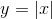, what is the correct order, from least to greatest, for the following expressions?

a)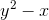b)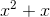c)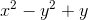Possible Answers:

a,b,c

a,c,b

b,a,c

c,b,a

c,a,b

Correct answer:

c,b,a

Explanation:

The easiest approach to this problem is to pick a value for X, and plug it in.

If we use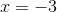, then then expression

a)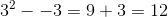.

b)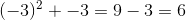c)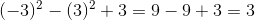The order from least to greatest is C,B,A

### Example Question #1 : Number Lines

Put the following numbers in order from least to greatest: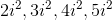Possible Answers: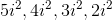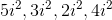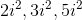Correct answer:Explanation: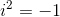, remembering that, then the most negative number is first, the least negative is last.

Therefore,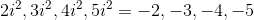.

Thus when we put them in order we get,### Example Question #93 : Number Theory

What are integers?

Possible Answers:

Positive whole numbers only.

Just.

Any number that is real.

Positive, negative whole numbers and.

Negative whole numbers only.

Correct answer:

Positive, negative whole numbers and.

Explanation:

Integers are values you would find on a number line.

They are whole numbers that exist in positive and negative values. What divides them is the number.

So integers are essentially positive, negative whole numbers and.

### Example Question #94 : Number Theory

Which of the following numbers is betweenand?

Possible Answers: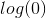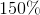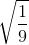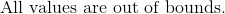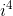Correct answer:Explanation:

The answer will lie in the range between negative one and a half.  Convert all the values given to integer or fractional form.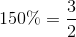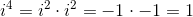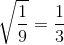is undefined.

Of all the possible answers,will fall between the given range.

The answer is:### All Algebra II Resources